Tutorial 12 – Decorators in Python

Welcome to the twelfth tutorial of Selenium-Python series! We will study about Decorators in python.

What you will Learn:
• Decorators
• Conclusion

Decorators
We have already used a decorator “abstractmethod’ in our previous article. To understand decorators further, let us create a simple ‘Divide’ class that has a method to divide 2 numbers. To call the function we create the object & print the result of division, see below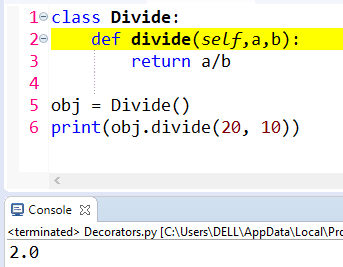Figure 1

However if we divide by 0, we get an expected error: “ZeroDivisionError: division by zero”, see below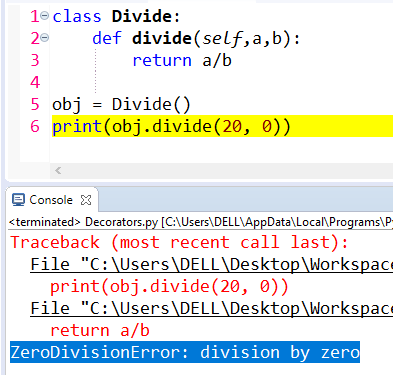Figure 2

We have already seen how to handle above type of errors in our ‘exception handling’ tutorial https://www.selenium-tutorial.com/blog/169026/selenium-python-tutotrial-exception-handling

There is another feature in python where we can add the functionality to an existing code. To add the functionality, we will create a simple function outside the class, see line 1 below. This ‘func’ is an argument over here.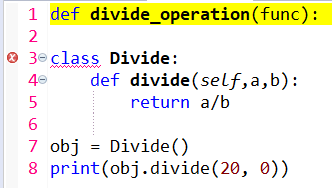Figure 3

Inside this function we will create another function (viz function inside a function). This function will accept ‘a’ & ‘b’, see below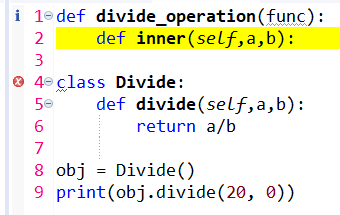Figure 4

Next we will write a print statement as see below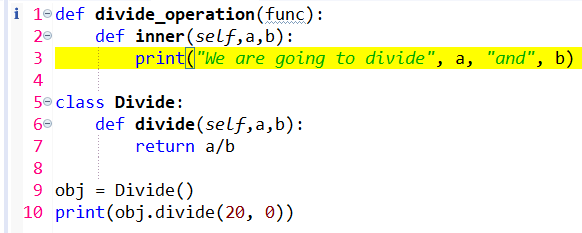Figure 5

Now we will put an ‘if’ condition over here, see lines 4 & 5 below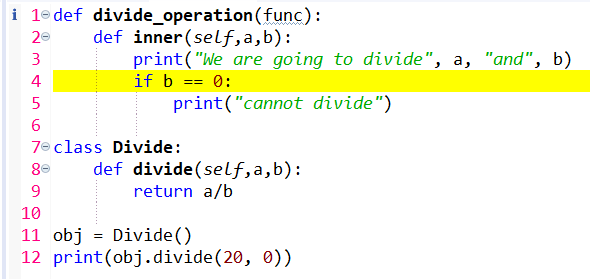Figure 5

So if b is 0 than we will quit the inner function, see line 6 below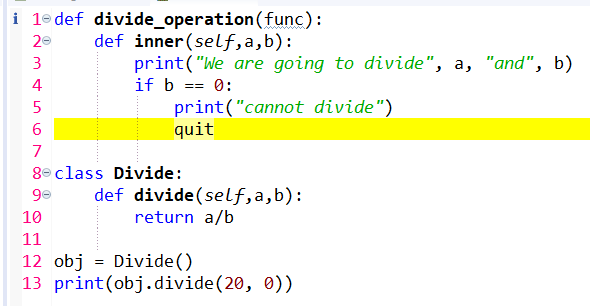Figure 6

Now how will this function run? To run this function, we add a decorator, see line number 6 below. So what does this decorator do? It will run the inner function (line 2). The ‘divide’ function (line 10) will be passed to the ‘func’ argument of divide_operation function (line 1).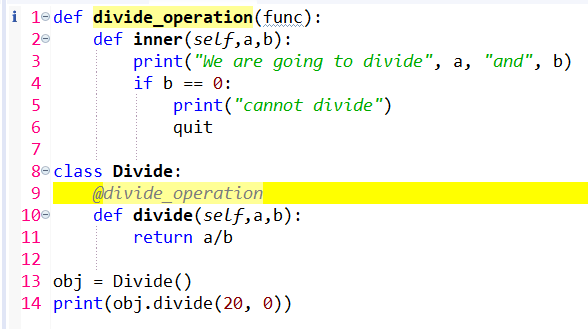Figure 7

Let us catch the result in a ‘result’ variable, see line 14 below & than print the result. When we run the program, we get an error: “TypeError: 'NoneType' object is not callable”, see below.
‘NoneType’ is the type of the 'None' object which represents a lack of value, for example, a function that does not explicitly return a value will return 'None'. So basically we are calling a value that is not callable. Hence we are not able to catch any result in line 14.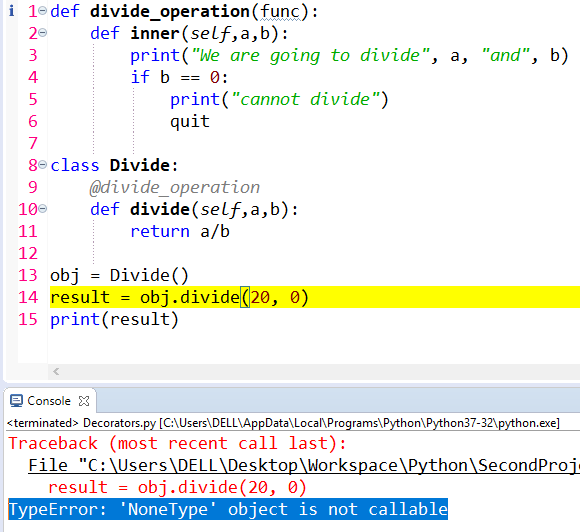Figure 8

To fix this error, the ‘inner’ function should return us the ‘func’, see line 7 below. However the program still gives us the same error: “TypeError: 'NoneType' object is not callable”. The reason is ‘divide_operation’ function is not returning something.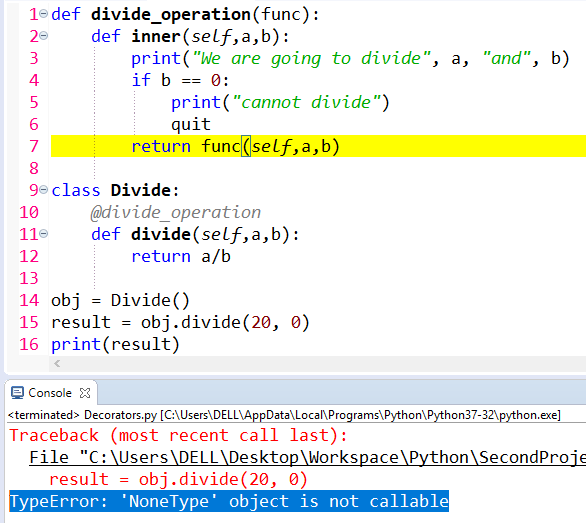Figure 9

So, see line 8 below. We are returning ‘inner’ function. When we run, we now see the o/p without any error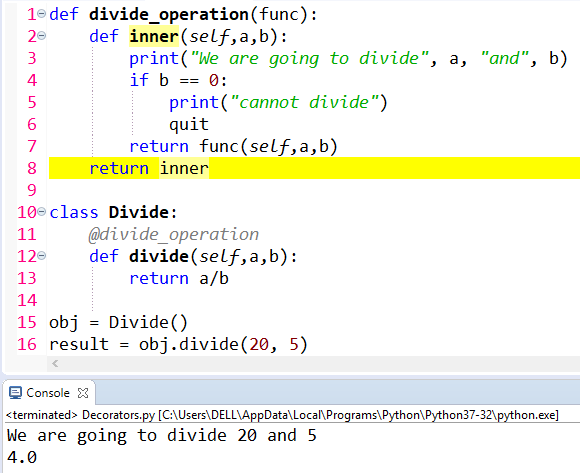Figure 10

Now if we try to divide by 0, we should not get ‘ZeroDivisionException’, however we are getting an exception, see below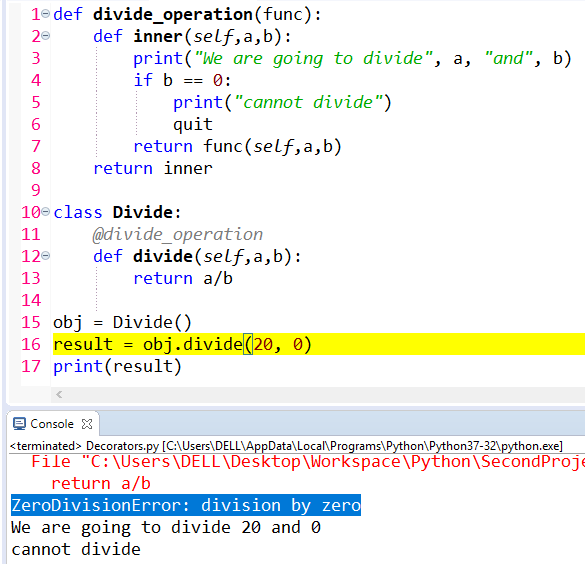Figure 11

The reason being, we should use quit() instead of quit, see line 6 below. Now when you run, there is no exception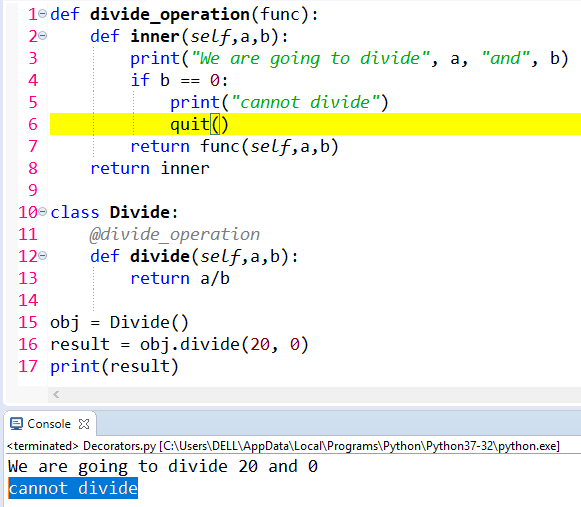Figure 12

So this decorator adds the functionality to the code.

Conclusion
In this tutorial we have studied about decorators in python. Thank you for reading!